Examples

Chapter 6 Class 12 Application of Derivatives
Serial order wise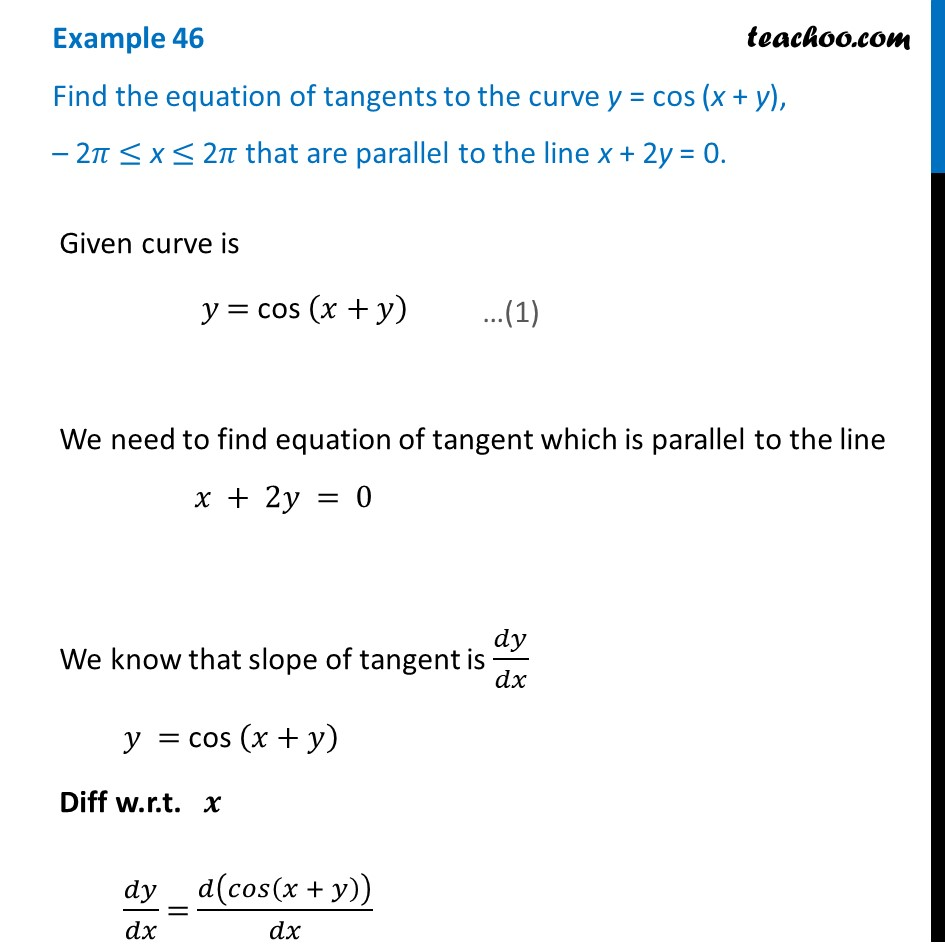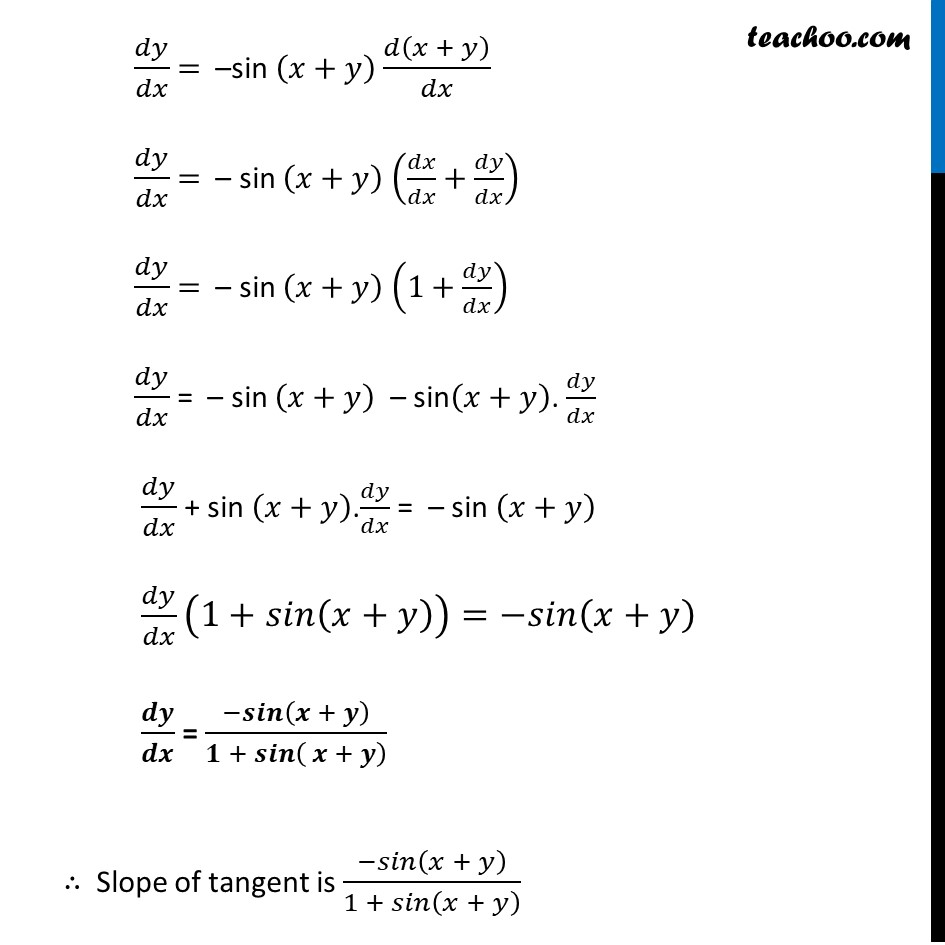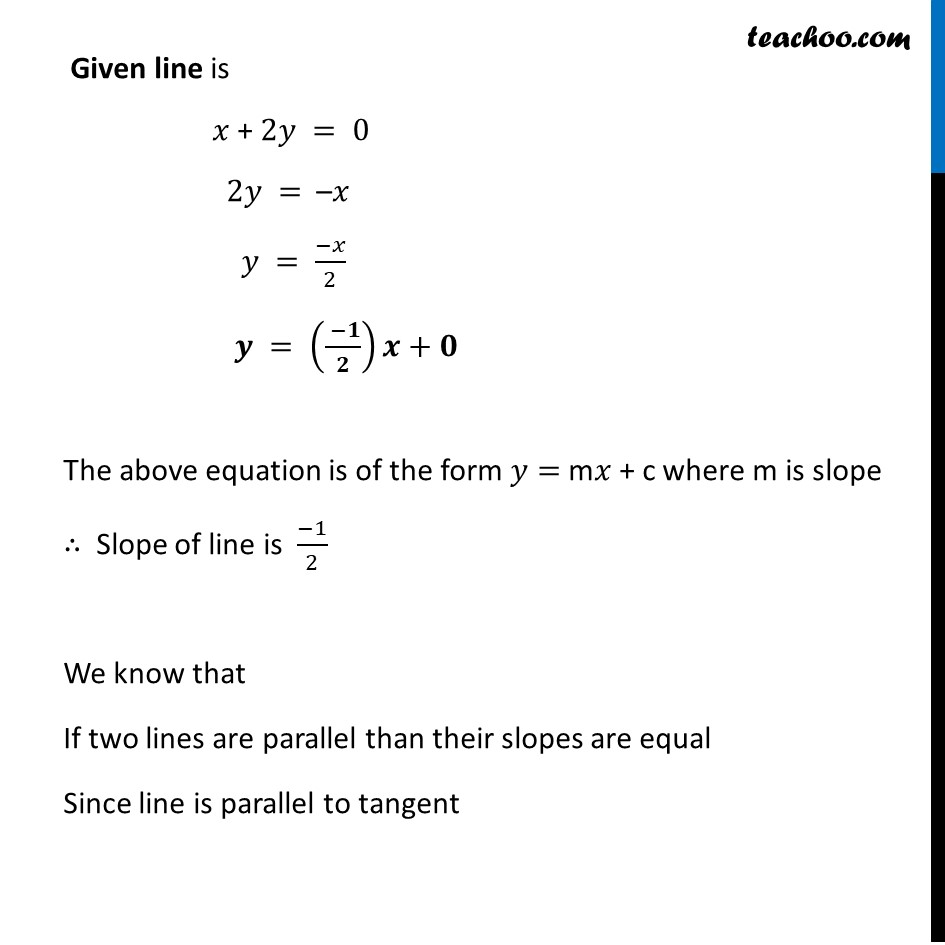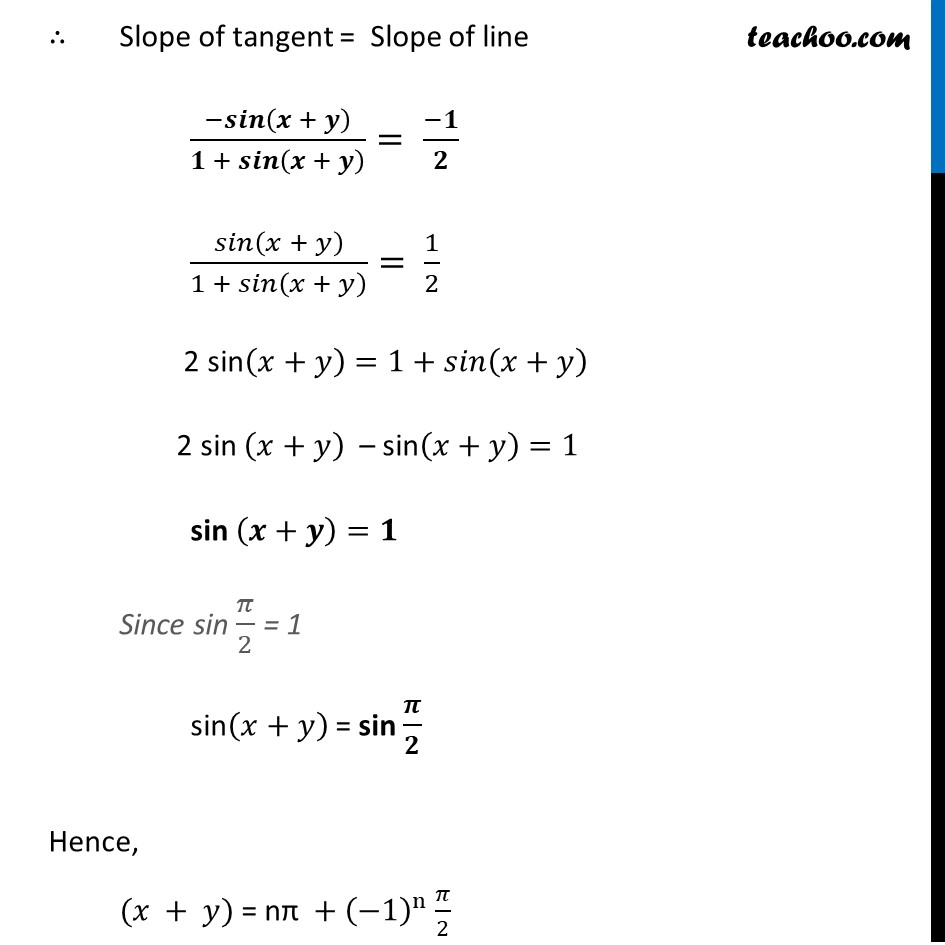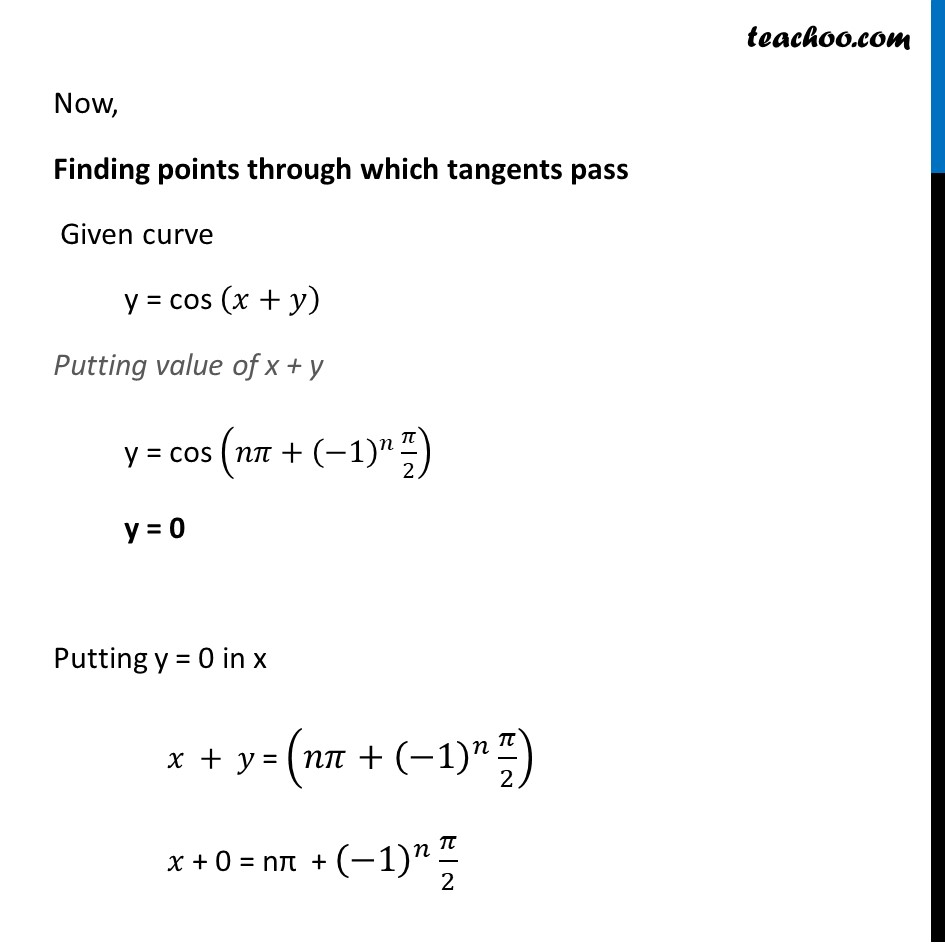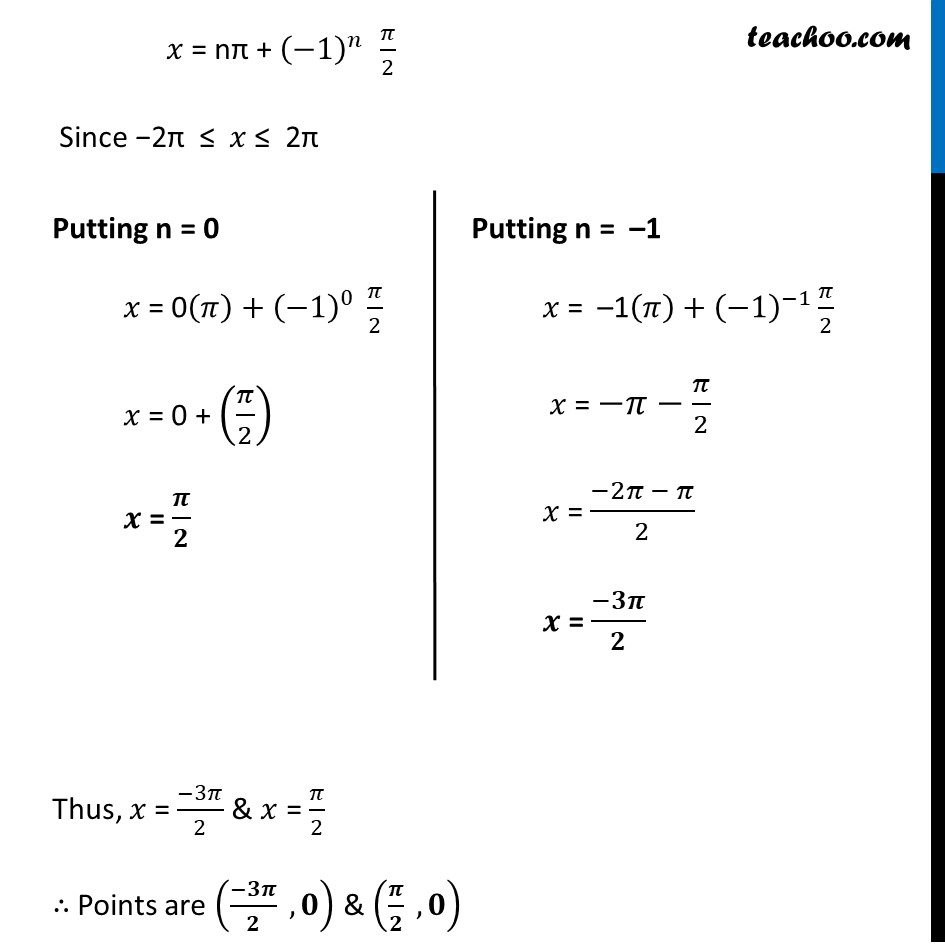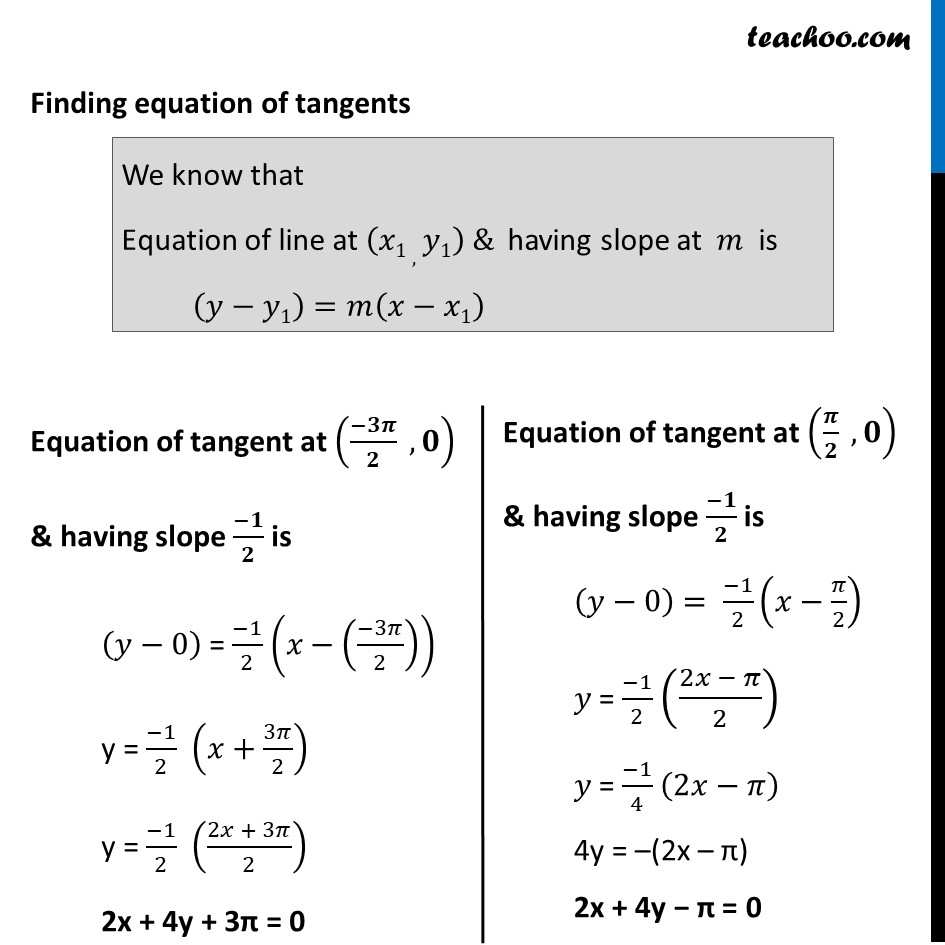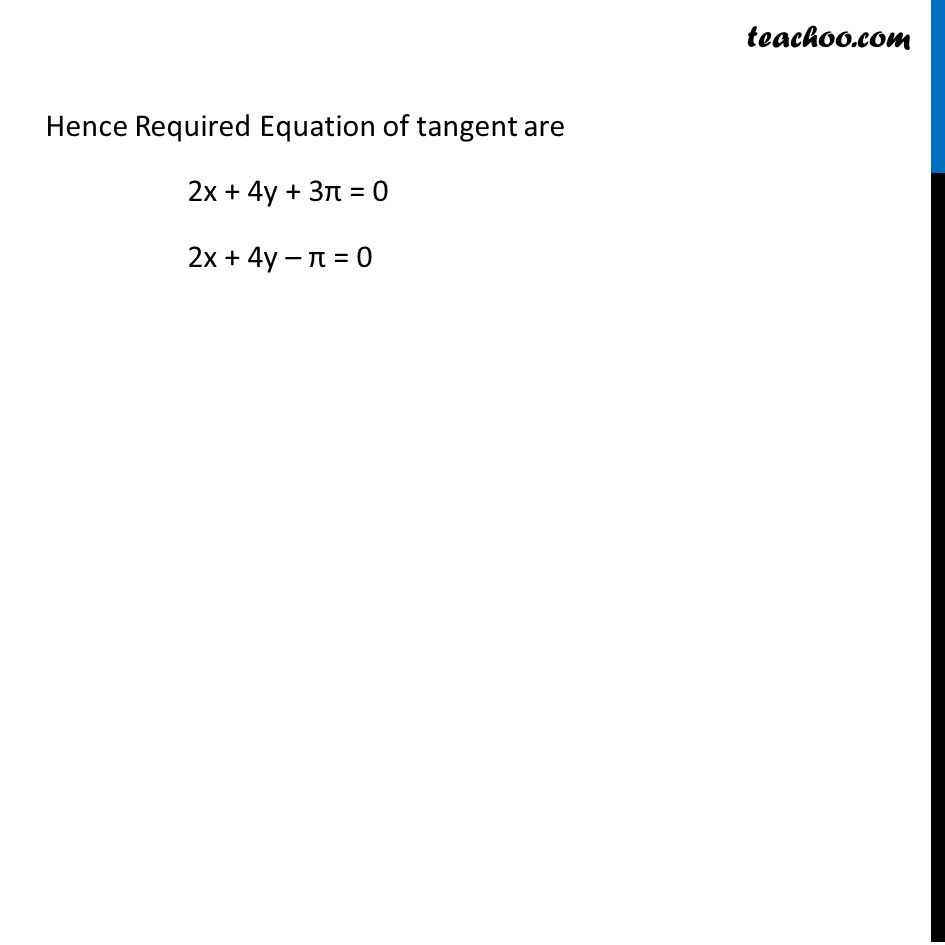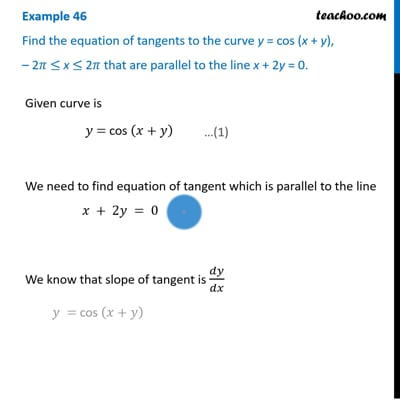This video is only available for Teachoo black users

Maths Crash Course - Live lectures + all videos + Real time Doubt solving!

### Transcript

Example 46 Find the equation of tangents to the curve y = cos (x + y), – 2𝜋 ≤ x ≤ 2𝜋 that are parallel to the line x + 2y = 0. Given curve is 𝑦 = cos (𝑥+𝑦) We need to find equation of tangent which is parallel to the line 𝑥 + 2𝑦 = 0 We know that slope of tangent is 𝑑𝑦/𝑑𝑥 𝑦 = cos (𝑥+𝑦) Diff w.r.t. 𝒙 𝑑𝑦/𝑑𝑥 = 𝑑(𝑐𝑜𝑠(𝑥 + 𝑦))/𝑑𝑥 𝑑𝑦/𝑑𝑥 = –sin (𝑥+𝑦) 𝑑(𝑥 + 𝑦)/𝑑𝑥 𝑑𝑦/𝑑𝑥 = – sin (𝑥+𝑦) (𝑑𝑥/𝑑𝑥+𝑑𝑦/𝑑𝑥) 𝑑𝑦/𝑑𝑥 = – sin (𝑥+𝑦) (1+𝑑𝑦/𝑑𝑥) 𝑑𝑦/𝑑𝑥 = – sin (𝑥+𝑦) – sin(𝑥+𝑦). 𝑑𝑦/𝑑𝑥 𝑑𝑦/𝑑𝑥 + sin (𝑥+𝑦).𝑑𝑦/𝑑𝑥 = – sin (𝑥+𝑦) 𝑑𝑦/𝑑𝑥 (1+𝑠𝑖𝑛(𝑥+𝑦))=−𝑠𝑖𝑛(𝑥+𝑦) 𝒅𝒚/𝒅𝒙 = (−𝒔𝒊𝒏(𝒙 + 𝒚))/(𝟏 + 𝒔𝒊𝒏( 𝒙 + 𝒚) ) ∴ Slope of tangent is (−𝑠𝑖𝑛(𝑥 + 𝑦))/(1 + 𝑠𝑖𝑛(𝑥 + 𝑦) ) Given line is 𝑥 + 2𝑦 = 0 2𝑦 = –𝑥 𝑦 = (−𝑥)/2 𝒚 = (( −𝟏)/𝟐)𝒙+𝟎 The above equation is of the form 𝑦= m𝑥 + c where m is slope ∴ Slope of line is (−1)/2 We know that If two lines are parallel than their slopes are equal Since line is parallel to tangent ∴ Slope of tangent = Slope of line (−𝒔𝒊𝒏(𝒙 + 𝒚))/(𝟏 + 𝒔𝒊𝒏(𝒙 + 𝒚) )= (−𝟏)/𝟐 𝑠𝑖𝑛(𝑥 + 𝑦)/(1 + 𝑠𝑖𝑛(𝑥 + 𝑦) )= 1/2 2 sin(𝑥+𝑦)=1+𝑠𝑖𝑛(𝑥+𝑦) 2 sin (𝑥+𝑦) – sin(𝑥+𝑦)=1 sin (𝒙+𝒚)=𝟏 Since sin 𝜋/2 = 1 sin(𝑥+𝑦) = sin 𝝅/𝟐 Hence, (𝑥 + 𝑦) = nπ + (−1)^n 𝜋/2 Now, Finding points through which tangents pass Given curve y = cos (𝑥+𝑦) Putting value of x + y y = cos (𝑛𝜋+(−1)^𝑛 𝜋/2) y = 0 Putting y = 0 in x 𝑥 + 𝑦 = (𝑛𝜋+(−1)^𝑛 𝜋/2) 𝑥 + 0 = nπ + (−1)^𝑛 𝜋/2 𝑥 = nπ + (−1)^(𝑛 ) 𝜋/2 Since −2π ≤ 𝑥 ≤ 2π Thus, 𝑥 = (−3𝜋)/2 & 𝑥 = 𝜋/2 ∴ Points are ((−𝟑𝝅)/𝟐 , 𝟎) & (𝝅/𝟐 , 𝟎) Putting n = 0 𝑥 = 0(𝜋)+(−1)^0 𝜋/2 𝑥 = 0 + (𝜋/2) 𝒙 = 𝝅/𝟐 Putting n = –1 𝑥 = –1(𝜋)+(−1)^(−1) 𝜋/2 𝑥 = −𝜋−𝜋/2 𝑥 = (−2𝜋 − 𝜋)/2 𝒙 = (−𝟑𝝅)/𝟐 Finding equation of tangents We know that Equation of line at (𝑥1 ,𝑦1) & having slope at 𝑚 is (𝑦−𝑦1)=𝑚(𝑥−𝑥1) Equation of tangent at ((−𝟑𝝅)/𝟐 , 𝟎) & having slope (−𝟏)/𝟐 is (𝑦−0) = (−1)/2 (𝑥−((−3𝜋)/2)) y = (−1)/2 (𝑥+3𝜋/2) y = (−1)/2 ((2𝑥 + 3𝜋)/2) 2x + 4y + 3π = 0 Equation of tangent at (𝝅/𝟐 , 𝟎) & having slope (−𝟏)/𝟐 is (𝑦−0)= (−1)/2 (𝑥−𝜋/2) 𝑦 = (−1)/2 ((2𝑥 − 𝜋)/2) 𝑦 = (−1)/4 (2𝑥−𝜋) 4y = –(2x – π) 2x + 4y − π = 0 Hence Required Equation of tangent are 2x + 4y + 3π = 0 2x + 4y – π = 0